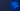posts# Efficient code vs string concatenation. Solve This Java™ Snippet!

###### 5.4

Let's have some fun with Java code! Here is a code snippet:

``````  int n = 100;

String s = "1";

for(int i = 0; i < n; i++)

s = s + 1;

``````

A cycle that repeats an ‘n’ number of times and adds ‘1’ to an ‘s’ string with an assigned value of ‘1.’

## Can you assess the algorithmic complexity?

This may not be as simple as it seems.

It may look like doing ‘n’ operations means O(n) complexity. Well, study the operation precisely. The thing you should notice is that it’s a concatenation of a string accumulator and a string representation of an integer. At each iteration of the loop, ‘s’ grows:

``````1

11

111

....

``````

Since Java strings are immutable, growing means copying all previous contents. The number of copied characters will be

(1) + (1 + 1) + (1 + 1 + 1) + … ,

which is a well-known sum of an arithmetic progression calculated as (n^2-n)/2. It means time complexity will be quadratic: O(n^2).

Let’s check what happens under the hood and how the benchmarks behave. This explanation is relevant for Java versions newer than 8, where such code is more efficient. There are comprehensive materials online that explain the Java 8 situation in depth.1 All examples are made with Liberica JDK.

If we inspect the bytecode targeted to Java 9+, there will be fragments like the `invokedynamic` call for string concatenation:

``````  14: invokedynamic #4,  0              // InvokeDynamic #0:makeConcatWithConstants:(Ljava/lang/String;)Ljava/lang/String;

``````

and

``````BootstrapMethods:

0: #38 REF_invokeStatic java/lang/invoke/StringConcatFactory.makeConcatWithConstants:(Ljava/lang/invoke/MethodHandles\$Lookup;Ljava/lang/String;Ljava/lang/invoke/MethodType;Ljava/lang/String;[Ljava/lang/Object;)Ljava/lang/invoke/CallSite;

``````

But basically, the default behavior is similar to what see for Java 8 target when `javac` (the primary Java compiler) generates concatenation code equivalent to

``````  s = new StringBuilder().append(s).append(1).toString();

``````

What does interest us the most in this example? The JVM performance of this code, and whether it’s good enough. Now we’ll run some tests.

``````@State(Scope.Thread)

public class ConcatBench {

@Param({"100", "400"})

int n;

@Benchmark

public String chain() {

String s = "1";

for(int i = 0; i < n; i++)

s = s + 1;

return s;

}

@Benchmark

public String sameSB() {

String s = "1";

StringBuilder sb = new StringBuilder(s);

for(int i = 0; i < n; i++)

sb.append(1);

return sb.toString();

}

@Benchmark

public String newSB() {

String s = "1";

for(int i = 0; i < n; i++) {

s = new StringBuilder().append(s).append(1).toString();

}

return s;

}

}

``````

This example also has a third code variant where `StringBuilder` is created outside of the loop.

If we target `javac` to Java 16 and run the benchmark on JDK 16, we get the following:

``````Benchmark           (n)        Score        Error  Units

ConcatBench.chain   100   940733.514 ±  39773.301  ops/s

ConcatBench.newSB   100   867987.506 ±  10770.221  ops/s

ConcatBench.sameSB  100  4937177.165 ± 166843.186  ops/s # 5.2x faster than chain

ConcatBench.chain   400   128595.073 ±    819.279  ops/s # 7.3x slower than n=100

ConcatBench.newSB   400   127075.294 ±   1383.764  ops/s

ConcatBench.sameSB  400  1403760.812 ±  37459.769  ops/s # 3.5x slower than n=100, 11x faster than chain

``````

When ‘n’ grows by four times, the code variant where `StringBuilder` is reused slows down by 3.5x (close to linear), while the variant with re-created `StringBuilder` slows down by 7.3x. The exact quadratic slowdown would be 16-fold. However, there are additive costs and optimizations for data copying (see `StubRoutines::jbyte_disjoint_arraycopy` in the OpenJDK code).

As an experiment, you can run the benchmarks on your hardware. Try playing with `javac` and JDKs and compare 7, 8, 11, and 16 bytecode running on JDK 7, 8, 11, and 16.

## In short, prepare for a higher cost

The complexity will be quadratic — expressed as a sum of an arithmetic sequence to n terms — and it will be in the same proportion for both the number of operations and memory. The reason is string concatenation, an infamous Java caveat.

Isn’t performance in the JVM exciting? Would you like to see more of such posts? Subscribe to our newsletter and get informed about new articles first!